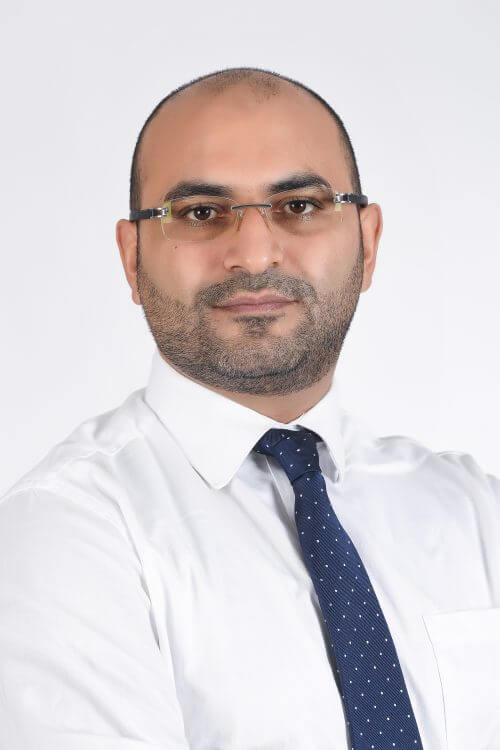Dr. Mohamed Kamel Riahi Assistant Professor
Telephone +971 2 312 3563
Bio
Education
Teaching Areas
Research Interests
Bio

### Assistant Professor, Department of Mathematics

Dr. Mohamed K. Riahi earned his PhD in Applied Mathematics from Pierre et Marie Curie University, Paris, France. He has worked as a postdoc research associate in the Department of Mathematical Sciences at New Jersey Institute of Technology, USA, and in INRIA-Saclay Palaiseau France as a postdoc researcher, with DeFI Team.

Dr. Riahi is an expert in scientific computing and numerical analysis related to partial differential equations. His research includes and is not limited to: space- time-domain decomposition methods, finite element method, integral equations method, numerical optimization, iterative method, preconditioning technique, shape optimization, optimal control problem, high-performance computing, and parallel programming.

He applies his research on waves related problems (acoustics and electromagnetism), fluid-structure interaction, heat and mass transfer, and inverse problems.

Dr. Riahi is serving as referee for several peer-reviewed applied mathematical journals.

Education
• PhD in Mathematical Sciences
• MSc In Applied Mathematics
Teaching Areas
• Calculus
• Optimization
• Advanced Numerical Methods (Master's degree)
• Numerical Analysis and Scientific computing
• Partial Differential Equations
Research Interests
• Partial differential equations
• Finite element methods
• Finite difference methods
• Integral equations
• Numerical analysis
• Non-linear optimization
• Mathematical physics/engineering
• Reaction diffusion systems
• Wave propagation
• Electromagnetism
• Fluid mechanics
• Heat transfer
• NMR
• Robust solvers
• Domain decomposition method
• Parareal method
• High-performance computing and parallel programming
• Inverse problems
• Optimal control
• Shape optimization
• Parameters identification
• Numerical analysis and scientific computing inverse problems
• Optimization and optimal control theory
• Computational fluid mechanics
• Computational physics
• Computational electromagnetism
• Numerical solution for PDEs.